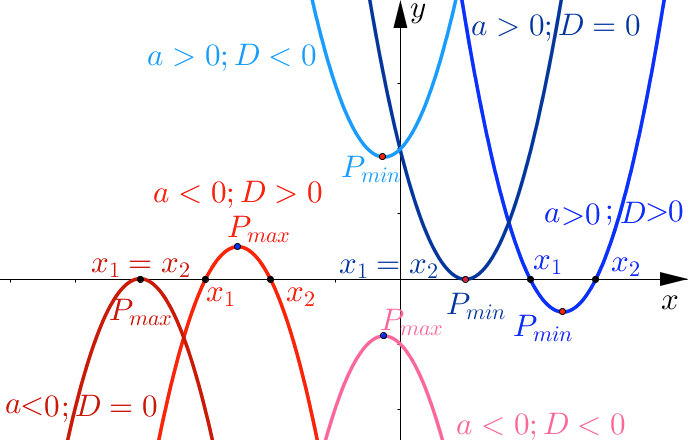Keywords:
$a{x}^{2}+bx+c=0$

${x}_{1,2}=\frac{-b±\sqrt{{b}^{2}-4ac}}{2a}$

Factors of a a quadratic equation:

$a{x}^{2}+bx+c=a\left(x-{x}_{1}\right)\left(x-{x}_{2}\right)$

Vieta's formulas:

Discriminant:

$D={b}^{2}-4ac$
 two different real solutions one real solution no real solution (two complex conjugate solutions)

$y=a{x}^{2}+bx+c$$P\left(-\frac{b}{2a},-\frac{{b}^{2}-4ac}{a}\right)$